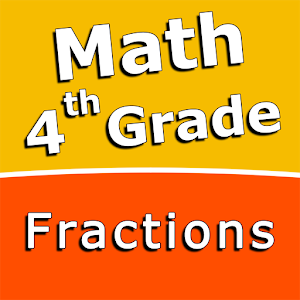\$2.99

# Android Giveaway of the Day - Fourth grade Math skills - Fractions

A collection of calculation tools and math-based games.
\$2.99 期限切れ
ユーザーの評価: 1 (100%) 0 (0%)

このgiveawayオファーはすでに期限が切れています。Fourth grade Math skills - Fractionsが今紹介されています。

Intuitive interface powered by handwriting input and a choice of three fun and engaging mini-games in addition to a regular math trainer mode makes our app stand out from the crowd of generic math learning apps.

With Fourth grade Math skills - Fractions you can practice and improve the following math skills:
- Add fractions with like denominators
- Subtract fractions with like denominators
- Add mixed numbers with like denominators
- Subtract mixed numbers with like denominators
- Add fractions with unlike denominators
- Subtract fractions with unlike denominators
- Add fractions with denominators of 10 and 100
- Add mixed numbers with unlike denominators
- Subtract mixed numbers with unlike denominators
- Multiply fractions by one-digit whole numbers

Choose between four different modes:
- Math Trainer
- Math Shot mini-game
- Math Gravity mini-game
- Math Trick Shot mini-game

Sergey Malugin

Education

1.0.0

11M

Everyone

4.0 and up

## コメント Fourth grade Math skills - Fractions

Thank you for voting!